# PSAT Math : How to find a solution set

## Example Questions

2 Next →

### Example Question #769 : Psat Mathematics

Which of the following is true of the solution set of the equation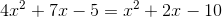?

The solution set comprises one rational number.

The solution set comprises two rational numbers.

The solution set comprises two irrational numbers.

The solution set comprises one irrational number.

The solution set comprises two imaginary numbers.

The solution set comprises two imaginary numbers.

Explanation:

First, since the equation is quadratic, put it in standard form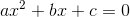as follows: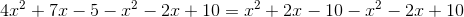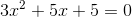To determine the nature of the solution set, evaluate discriminant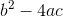for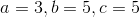: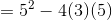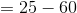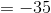The discriminant is negative, so the solution set comprises two imaginary numbers.

2 Next →